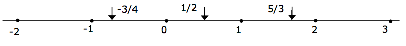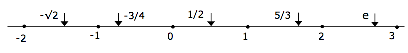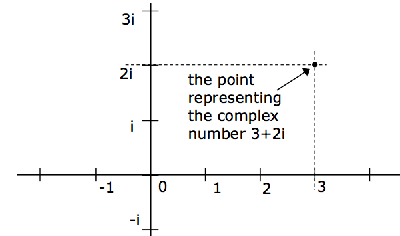# Divine Neutrality, Blog. Science, Philosophy

## Domains of Conception

March 19th, 2016

### The Borders of UnderstandingMy molecular biologist friend, Barry Bowman, brewed up this stimulating thought: One can understand much about nature without mathematics. Can one understand quantum mechanics only through its arcane mathematics?  Can one not comprehend quantum physics other than through its mathematical expression?

To explore the thought some agreement is needed on the meaning of understanding! I offer, for our data bank of shared images of nature, the following on mathematical understanding.

There exists counting because thingness is a property of nature; there are things in the world; in particular there are things that can be classified by sameness. You can count them. Eventually counting became indispensible for the pursuit of everyday affairs. Six shells for those two axe heads.

An understanding of integers derived from the needs of human interaction; of commerce. Those same needs brought about the notion of addition. Two shells plus four shells make up the six I need. The interesting thing about addition is that it always works; you add two numbers and you get another number.

The exigencies of business produced subtraction. It is the inverse of addition. If the merchant had six and two are removed he has four left. The remainder is four because adding two to four yields six. The addition is inverted. The remarkable thing about subtraction was this: It didn’t always work. Four minus six did not produce another number! It produced a meaningless thing. By ‘number’ was meant what we now call a positive integer. At the start of mathematics nothing else was known.

Imagine the brilliance of the man who gave meaning to a meaningless notion; to 4 minus 6! He said, “Let us accept ‘minus 2′ as a form of number – like 2 itself”. He invented negative numbers. So the practice of counting gave rise to a new kind of thing. Negative numbers acquired physical meaning: the number lacking. A feature of nature took mathematical expression.

To complete the list of all integers there is one labeled zero. The concept of nothing has a mathematical representation. This subtle and critical fact was realized in the West only about 1000 years ago, long after counting began. The counting numbers grew into all the integers; the positive ones, zero and all of the negative ones.

In the course of civilization another arithmetic operation found use. Multiplication. Adding a number to itself many times. 3×2=6. Very useful in the business of living. Five nails in every post and there are seven posts so we’ll need 35 nails. In ancient times very few people must have known the multiplication table that every school child learns today.

Again this operation – multiplication – ‘always works’. The product of two integers is, itself, another integer. But given an operation you can conceive of its inverse; something that will undo it. The inverse of multiplication is division. What number is it, such that when you multiply it by 7, you get 35? We write this as 35/7. A slash denotes division. And the answer is 5 because 5×7=35.

Like the previous inverse operation, this inverse operation also ‘didn’t always work’. It produced answers outside the realm of familiar mathematical objects. Although it is true that 6/3 has a meaning – the integer, 2, – no meaning was attached to 5/3. It wasn’t among the known universe of arithmetical objects.

The essential association to nature that allows the concept to have meaning is this: besides countable objects there are continuous domains in nature. A hectare of land. Or a length of line. Five such lengths might be divided into three parts. Fractions – ratios, rational numbers – relate to the partitioning of a continuous domain.

And so we acquire the idea of the number line. The universe of all that we call number can be matched to points on this line. Any conceivable ratio of integers is a number. Because an integer divided by 1 is the integer itself, the integers are, themselves, rationals. Here’s the thought schematically:Any ratio of integers – a rational number – has a place on this number line.

Soon, the exigencies of the civilization process brought forth another mathematical operation: squaring a number. Multiplying it by itself. This operation was useful in the design of structures. Again it always worked. Squaring a rational number always produced another rational number. 32 = 9, (2/3)2 = 4/9. But the inverse of this operation – getting the square root, finding the number whose square is given – didn’t always work. The square root of 9, written √9, is 3 and √(4/9) is 2/3; numbers within our conception of what numbers should be. But what rational number is the square root of 2 (written √2)?

“There are more things in heaven and earth, Horatio, than are dreamt of in your philosophy,” says Shakespeare’s Hamlet. And, indeed, there is no rational number that is the square root of 2. The value of √2 can be approximated by this rational number: 7/5. The rational number, 71/50, approximates it even closer. But the process never stops; no rational number exists that equals √2.

The reader must surely thirst for the proof of this assertion. I needn’t give it here. She may google “proof square root 2 irrational” to retrieve a proof. The key issue for us is this: an inverse operation led us to the existence of elements – irrational numbers – not seen in our earlier universe of thought.

You might think that by placing a dot on paper for every conceivable rational number you would soon take up every point on a line. But it isn’t true. In fact, most of the points on the line are not representable by rational numbers. So we must expand our concept of number to embrace the irrationals; numbers which cannot be expressed as ratios of integers. The number line now includes these.Perhaps the most important irrational numbers are π and e. They pervade every field of scientific and engineering endeavor. Worldly activity and mathematical ingenuity go hand in hand.

The square root operation produced yet another realm of number. This comes about because the square of any number is always positive. So the square root of a negative number has no meaning within the constellation of numbers on the number line. There are two square roots of 4; -2 and +2 because either one multiplied by itself yields 4. But there is no number that when multiplied by itself yields -4. In symbols, √(-4) = 2√(-1), written as 2i, corresponds to no point on the number line. Customarily the letter i is used to represent √(-1). It is the unit of what came to be called ‘imaginary numbers’. The points on the number line – all rationals plus all irrationals – are called ‘real’ numbers.

In the early nineteenth century it became clear to mathematicians that the domain of numbers must be enlarged to include both real and imaginary ones. The domain of numbers has expanded to all the points in a plane. A complex number has a real and an imaginary part as shown in the figure.Mathematical ideas involving imaginary numbers blossomed. The number i turned out to be a useful tool of computation. But it was not required in any portrayal of the physical world. None of the mathematical statements describing the laws of physics had an i in it. Only real numbers were needed to describe the real world. But in the early 20th century that changed. It was discovered that describing the real world did, indeed, require i! The key equation governing the many quantum phenomena that were making themselves apparent is the Schroedinger equation. And that has an i in it. To characterize reality imaginary numbers are needed!

Now what of the prospect of new arithmetic domains yet to be uncovered by some future inverse operation. It can be shown that there are none. Every arithmetic operation on the complex numbers always produces other complex numbers. All operations work!

At a conference dinner many years ago I had the good fortune to sit next to Shiing-shen Chern, a highly esteemed mathematician. I mentioned to him the story of inverse operations leading to new mathematical domains. I was bothered that it should all culminate in complex numbers; nothing beyond these will ever be needed; that the process should just come to a stop right there! “I find it very unsatisfying,” said I.

With the wisdom befitting the sage that he was Chern answered, “I find it very satisfying.”

### Comments

1. 1

I hope Barry Bowman likes your very clear explanation, although you didn’t go on to explain the connection with Quantum Mechanics.

But the enlargement of the real numbers doesn’t stop with i. While the complex numbers are the largest field with all the properties we love — including commutativity a x b = b x a, and associativity (a x b) x c = a x (b x c) — if we are willing to give up commutativity we get quaternions (with 3 square roots of -1) and if we are also willing to give up associativity we get the octonians or Cayley algebra (with 7 square roots of -1). The quaternions have the same algebra as the 2×2 unit matrix plus the three Pauli matrices, very important in physics. The octonians are connected with the five exceptional Lie groups G2, F4, E6, E7, E8, which may be relevant for superstring theory, and thus also possibly relevant for physics.

- Joel Primack @